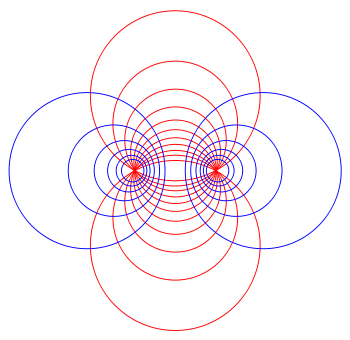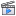# Linear system of divisorsA linear system of divisors algebraicizes the classic geometric notion of a family of curves, as in the Apollonian circles.

In algebraic geometry, a linear system of divisors is an algebraic generalization of the geometric notion of a family of curves; the dimension of the linear system corresponds to the number of parameters of the family.

These arose first in the form of a linear system of algebraic curves in the projective plane. It assumed a more general form, through gradual generalisation, so that one could speak of linear equivalence of divisors D on a general scheme or even a ringed space (X, OX).

A linear system of dimension 1, 2, or 3 is called a pencil, a net, or a web.

## Definition

Given the fundamental idea of a rational function on a general variety V, or in other words of a function f in the function field of V, divisors D and E are linearly equivalent ifwhere (f) denotes the divisor of zeroes and poles of the function f.

Note that if V has singular points, 'divisor' is inherently ambiguous (Cartier divisors, Weil divisors: see divisor (algebraic geometry)). The definition in that case is usually said with greater care (using invertible sheaves or holomorphic line bundles); see below.

A complete linear system on V is defined as the set of all effective divisors linearly equivalent to some given divisor D. It is denoted |D|. Let L(D) be the line bundle associated to D. In the case that V is a nonsingular projective variety the set |D| is in natural bijection withand is therefore a projective space.

A linear systemis then a projective subspace of a complete linear system, so it corresponds to a vector subspace W ofThe dimension of the linear systemis its dimension as a projective space. Hence.

Since a Cartier divisor class is an isomorphism class of a line bundle, linear systems can also be introduced by means of the line bundle or invertible sheaf language, without reference to divisors at all. In those terms, divisors D (Cartier divisors, to be precise) correspond to line bundles, and linear equivalence of two divisors means that the corresponding line bundles are isomorphic.

## Linear system of conics

For example, the conic sections in the projective plane form a linear system of dimension five, as one sees by counting the constants in the degree two equations. The condition to pass through a given point P imposes a single linear condition, so that conics C through P form a linear system of dimension 4. Other types of condition that are of interest include tangency to a given line L.

In the most elementary treatments a linear system appears in the form of equationswith λ and μ unknown scalars, not both zero. Here C and C are given conics. Abstractly we can say that this is a projective line in the space of all conics, on which we takeas homogeneous coordinates. Geometrically we notice that any point Q common to C and C is also on each of the conics of the linear system. According to Bézout's theorem C and C will intersect in four points (if counted correctly). Assuming these are in general position, i.e. four distinct intersections, we get another interpretation of the linear system as the conics passing through the four given points (note that the codimension four here matches the dimension, one, in the five-dimensional space of conics). Note that of these conics, exactly three are degenerate, each consisting of a pair of lines, corresponding to theways of choosing 2 pairs of points from 4 points (counting via the multinomial coefficient, and accounting for the overcount by a factor of 2 thatmakes when interested in counting pairs of pairs rather than just selections of size 2).

### Applications

A striking application of such a family is in (Faucette 1996) which gives a geometric solution to a quartic equation by considering the pencil of conics through the four roots of the quartic, and identifying the three degenerate conics with the three roots of the resolvent cubic.

### ExampleType I linear system, (Coffman).

For example, given the four pointsthe pencil of conics through them can be parameterized aswhich are the affine combinations of the equationsandcorresponding to the parallel vertical lines and horizontal lines; this yields degenerate conics at the standard points ofA less elegant but more symmetric parametrization is given byin which case inverting a () interchanges x and y, yielding the following pencil; in all cases the center is at the origin:

•hyperbolae opening left and right;
•the parallel vertical lines(intersection point at [1:0:0])
•ellipses with a vertical major axis;
•a circle (with radius);
•ellipses with a horizontal major axis;
•the parallel horizontal lines(intersection point at [0:1:0])
•hyperbolae opening up and down,
•the diagonal lines(dividing byand taking the limit asyields)
(intersection point at [0:0:1])
• This then loops around tosince pencils are a projective line.

In the terminology of (Levy 1964), this is a Type I linear system of conics, and is animated in the linked video.

### Classification

There are 8 types of linear systems of conics over the complex numbers, depending on intersection multiplicity at the base points, which divide into 13 types over the real numbers, depending on whether the base points are real or imaginary; this is discussed in (Levy 1964) and illustrated in (Coffman).

## Other examples

The Cayley–Bacharach theorem is a property of a pencil of cubics, which states that the base locus satisfies an "8 implies 9" property: any cubic containing 8 of the points necessarily contains the 9th.

## Linear systems in birational geometry

In general linear systems became a basic tool of birational geometry as practised by the Italian school of algebraic geometry. The technical demands became quite stringent; later developments clarified a number of issues. The computation of the relevant dimensions the Riemann–Roch problem as it can be called can be better phrased in terms of homological algebra. The effect of working on varieties with singular points is to show up a difference between Weil divisors (in the free abelian group generated by codimension-one subvarieties), and Cartier divisors coming from sections of invertible sheaves.

The Italian school liked to reduce the geometry on an algebraic surface to that of linear systems cut out by surfaces in three-space; Zariski wrote his celebrated book Algebraic Surfaces to try to pull together the methods, involving linear systems with fixed base points. There was a controversy, one of the final issues in the conflict between 'old' and 'new' points of view in algebraic geometry, over Henri Poincaré's characteristic linear system of an algebraic family of curves on an algebraic surface.

## Base locus

The base locus of a linear system of divisors on a variety refers to the subvariety of points 'common' to all divisors in the linear system. Geometrically, this corresponds to the common intersection of the varieties. Linear systems may or may not have a base locus – for example, the pencil of affine lineshas no common intersection, but given two (nondegenerate) conics in the complex projective plane, they intersect in four points (counting with multiplicity) and thus the pencil they define has these points as base locus.

More precisely, suppose thatis a linear system of divisors on some variety. Consider the intersectionwheredenotes the support of a divisor, and the intersection is taken over all effective divisorsin the linear system. This is the base locus of(as a set, at least: there may be more subtle scheme-theoretic considerations as to what the structure sheaf ofshould be).

One application of the notion of base locus is to nefness of a Cartier divisor class (i.e. complete linear system). Supposeis such a class on a variety, andan irreducible curve on. Ifis not contained in the base locus of, then there exists some divisorin the class which does not contain, and so intersects it properly. Basic facts from intersection theory then tell us that we must have. The conclusion is that to check nefness of a divisor class, it suffices to compute the intersection number with curves contained in the base locus of the class. So, roughly speaking, the 'smaller' the base locus, the 'more likely' it is that the class is nef.

In the modern formulation of algebraic geometry, a linear systemof (Cartier) divisors on a varietyis viewed as a line bundleon. From this viewpoint, the base locusis the set of common zeroes of all sections of. A simple consequence is that the bundle is globally generated if and only if the base locus is empty.

## References

1. Grothendieck, Alexandre; Dieudonné, Jean. EGA IV, 21.3.
2. Hartshorne, R. 'Algebraic Geometry', proposition II.7.7, page 157
• Coffman, Adam, Linear Systems of Conics
• Faucette, William Mark (January 1996), "A Geometric Interpretation of the Solution of the General Quartic Polynomial", The American Mathematical Monthly, 103 (1): 51–57, CiteSeerX 10.1.1.111.5574, JSTOR 2975214
• P. Griffiths; J. Harris (1994). Principles of Algebraic Geometry. Wiley Classics Library. Wiley Interscience. p. 137. ISBN 0-471-05059-8.
• Hartshorne, R. Algebraic Geometry, Springer-Verlag, 1977; corrected 6th printing, 1993. ISBN 0-387-90244-9.
• Levy, Harry (1964), Projective and related geometries, New York: The Macmillan Co., pp. x+405
• Lazarsfeld, R., Positivity in Algebraic Geometry I, Springer-Verlag, 2004. ISBN 3-540-22533-1.